Next: About this document Up: The linear approximation to Previous: Background

## Exercises

1. Try out tangentline on the following examples. This part of the lab is intended to help you become more familiar with the concept of the tangent line to a function f(x) at a point x=a. Use the given values of a along with another value of your choice to find 3 tangent lines for each function. Also, for each function, plot the function and the three tangent lines on the same graph.
1.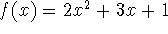with a = 2 and a = -3.
2.with a = 0 and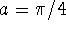.
3.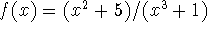with a = 2 and a = 1.
2. More generally, the graphs of two functions f(x) and g(x) are tangent at x=a if f(a) = g(a) and f'(a) = g'(a). For example, the functionsand g(x) = x are tangent at x=0, but the functions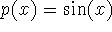and q(x) = x+1 are not tangent at x=0, even though they have the same slope, because p(0) = 0 and q(0) = 1 so the graphs of p and q don't intersect at x=0.

Suppose that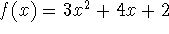and. Determine if the following statements are true or not.

1.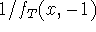is tangent to 1/f(x) at x=-1.
2.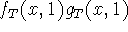is tangent to f(x)g(x) at x=1.
3.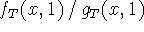is tangent to f(x)/g(x) at x=1.
4.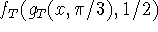is tangent to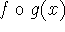at.

Sean O Anderson
Tue Sep 24 13:24:30 EDT 1996